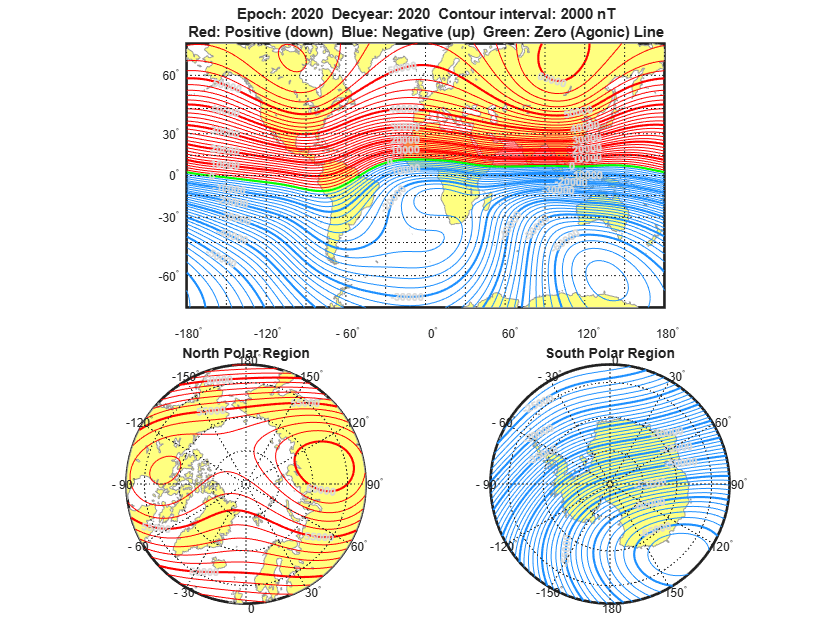# Visualize World Magnetic Model Contours for 2020 Epoch

This example shows how to visualize contour plots of the calculated values for the Earth's magnetic field using World Magnetic Model 2020 (WMM2020) overlaid on maps of the Earth.

The Mapping Toolbox™ is required to generate the maps of the Earth.

### Generate Values for Earth's Magnetic Field

Calculate these values for the Earth magnetic field. To implement the World Magnetic Model 2020 (WMM2020), use the `wrldmagm` function.

• X - North component of magnetic field vector in nanotesla (nT)

• Y - East component of magnetic field vector in nanotesla (nT)

• Z - Down component of magnetic field vector in nanotesla (nT)

• H - Horizontal intensity in nanotesla (nT)

• DEC - Declination in degrees

• DIP - Inclination in degrees

• F - Total intensity in nanotesla (nT)

Based on these `wrldmagm` inputs:

• model_epoch - Epoch of WMM model.

• decimal_year - Scalar value representing the decimal year within the epoch for which the data was generated.

```model_epoch = '2020'; decimal_year = 2020;```

For a given epoch and decimal year, use the following code to generate 13021 data points for calculating values of Earth's magnetic field using `wrldmagm`. To reduce overhead calculation, this model includes a MAT-file that contains this data for epoch 2020 and decimal year 2020.

Assume zero height.

`% height = 0;`

Geodetic Longitude value in degrees to use for latitude sweep.

`% geod_lon = -180:1:180;`

Geodetic Latitude values in degrees to sweep.

`% geod_lat = -89.5:.5:89.5;`

Loop through longitude values for each array of latitudes -89.5:89.5.

```% for lonIdx = size(geod_lon,2):-1:1 % for latIdx = size(geod_lat,2):-1:1```

To obtain magnetic parameters for each latitude and longitude value, use the `wrldmagm` function.

`% [xyz, h, dec, dip, f] = wrldmagm(height, geod_lat(latIdx),geod_lon(lonIdx), decimal_year, model_epoch); `

Store the results.

```% WMMResults(latIdx,1:7,lonIdx) = [xyz' h dec dip f]; % % end % end```

Load the saved data in the MAT-file.

```WMMFileName = 'astWMMResults_Epoch_2020_decyear_2020.mat'; load(WMMFileName);```

Read in the continent land areas for plot overlay using the Mapping Toolbox function, `shaperead`.

`landAreas = shaperead('landareas.shp','UseGeoCoords',true);`

### Plotting Earth's Magnetic Field Overlaid on Earth Maps

Load plot formatting data for each of the magnetic parameters.

`plotWMM = load('astPlotWMM.mat');`
```hX = figure; set(hX,'Position',[0 0 827 620],'Color','white') astPlotWMMContours( WMMResults, plotWMM, 1, landAreas, geod_lat, geod_lon, decimal_year, model_epoch)```Figure 1: North Component of Magnetic Field Vector, X (nT)

```hY = figure; set(hY,'Position',[0 0 827 620],'Color','white') astPlotWMMContours( WMMResults, plotWMM, 2, landAreas, geod_lat, geod_lon, decimal_year, model_epoch)```Figure 2: East Component of Magnetic Field Vector, Y (nT)

```hZ = figure; set(hZ,'Position',[0 0 827 620],'Color','white') astPlotWMMContours( WMMResults, plotWMM, 3, landAreas, geod_lat, geod_lon, decimal_year, model_epoch)```Figure 3: Down Component of Magnetic Field Vector, Z (nT)

```hH = figure; set(hH,'Position',[0 0 827 620],'Color','white') astPlotWMMContours( WMMResults, plotWMM, 4, landAreas, geod_lat, geod_lon, decimal_year, model_epoch)```Figure 4: Horizontal Intensity, H (nT)

```hDEC = figure; set(hDEC,'Position',[0 0 827 620],'Color','white') astPlotWMMContours( WMMResults, plotWMM, 5, landAreas, geod_lat, geod_lon, decimal_year, model_epoch)```Figure 5: Declination, DEC (deg)

```hDIP = figure; set(hDIP,'Position',[0 0 827 620],'Color','white') astPlotWMMContours( WMMResults, plotWMM, 6, landAreas, geod_lat, geod_lon, decimal_year, model_epoch)```Figure 6: Inclination, DIP (deg)

```hF = figure; set(hF,'Position',[0 0 827 620],'Color','white') astPlotWMMContours( WMMResults, plotWMM, 7, landAreas, geod_lat, geod_lon, decimal_year, model_epoch)```Figure 7: Total Intensity, F (nT)

`close (hX, hY, hZ, hH, hDEC, hDIP, hF)`